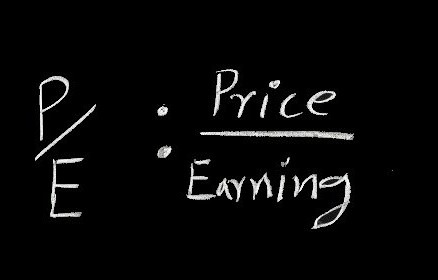Latest Articles
Home / Finance / Financial Market / Price-Earning Ratio Approach

# Price-Earning Ratio Approach

Price-Earning Ratio Approach.

The ratio is the ratio of price per share to earning per share is commonly known as the price-earning ratio. Much of the real world discussion of stock market valuation concentrates on the firm’s price-earning multiple. Earning a multiplier approach states that the price of the stock is equal to the product of its earnings and a multiplier. It implies that the price of a stock is the product of EPS and P/E multiplier of that stock.

Page Contents

# Price-Earning Ratio Approach

Price-earning (P/E) ratio approach postulates that the current market price of a stock can be determined as under:

P0 = E0×P0/E0

Where E, being the estimated earnings for the next twelve months, refers to the earnings used to calculate the P/E ratio. Therefore;

P0 = Estimated earnings × estimated P/E ratio

## Determinants of Price-Earning Ratio Approach

The constant-growth version of the dividend discount model estimates the price of the stock as follows:

P0 = D1 / (k-g)

By dividing both the sides of the equation by expected earnings (E1), we get:

P0/E1= [D1 / (k-g)]/E1

P0/E1= P0/E1

P0= [P0/E1] ×E1

Therefore, the determinants of the P/E ratio are;

1. The dividend payout ratio, DPS/EPS
2. Investor’s required rate of return, k
• The expected growth rate of dividend, g.

These can be discussed as:

• The higher the payout ratio, the higher the P/E ratio
• The higher the expected growth rate, the higher the P/E ratio
• The higher the required rate of return, the lower the P/E ratio.

## Example

Suppose, the payout ratio of a firm is .60. The required rate of return is 15 percent with an expected growth rate of 7 percent. If the expected earnings of the firm for the next year is Tk.10, what would be the price of the share of that firm?

P/E = [D1/E1]/k-g

= .60/.15-.07 = .60/.08 = 7.50

Therefore, the price for the stock is

P0 = [P/E] ×E1

= 7.50 × 10 = Tk. 75

If the investor’s required rate of return, k, is 12 per cent and growth rate is being 7 per cent;

P/E = .60/.12-.07 = .60/.05 = 12 and P0 = 12×10 = Tk. 120.

If the investor’s required rate of return, k, is 18 per cent and growth rate is being 7 per cent;

P/E = .60/.18 -.07 = .60/.11 = 5.45 and P0 = 5.45 ×10 = Tk. 54.50.

We can think of the value of the firm as the sum of the value of assets or the no-growth value of the firm plus the net present value of the future investments, the firm will make. The present value is called the present value of growth opportunities, PVGO. Therefore, the value of the firm is:

P0 = No-growth value per share + PVGO

P0 = [E1/k] + PVGO

Now recall that the dividends represent the earnings which are not retained for reinvestment purpose. The growth rate of dividends is, therefore, the ratio of earnings reinvested to book value of the firm like:

g = Reinvested earnings/ Book value

If earnings equal to the product of return on equity and book value (ROE × book value), the growth of dividend would become:

g = [Reinvested earnings/ Book value] × [Total earnings/ Book value]

g = [b × ROE]

Hence, substituting for expected dividends (D1) and growth of dividend (g) in the constant-growth version of DDM, we find the value of the firm as:

P0 = E1(1-b) / k – (ROE ×b)

Implying the P/E ratio, we get

P0 / E1= (1-b) / k– (ROE ×b)

Assume that risk-free rate is 7 %, risk premium (rm– rf) is also 5 %, and retention ratio is 40 %. Therefore,

rm =  rf + market risk premium = 7 % + 5% = 12%. For a company with beta (b) equals to 1, the required rate of return of the firm, k, will become equal to 12%. The return on equity (ROE) of that firm would also become equal to the expected return on the stock. Therefore, the growth of the firm would become:

g = .12 × .40 = .048 = 4.80%

P/E = (1-.40) / (0.12 – .048) = 8.33.

We may observe that one important implication of stock valuation model is that riskier stock will have lower P/E multiples which can be expressed as:

P/E= (1-b) / (k-g).

The riskier firm will have a higher required rate of return implying a higher value of k, therefore, P/E multiple will become lower.